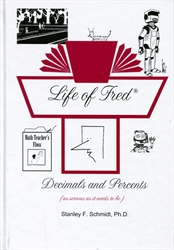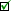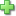# Life of Fred: Decimals and Percents

## (as serious as it needs to be)

Life of Fred
by Stanley F. Schmidt, Stanley F. Schmidt
Hardcover, 191 pages
Price: \$24.00
Used Price: \$13.00 (1 in stock)Condition Policy
• Number Systems
• Multiplying by Ten
• Functions and Inverse Functions
• Pi
• Multiplying Decimals
• Whole Numbers
• Squaring a Number
• Subtracting Mixed Units
• Sets and Subsets
• Union and Intersection of Sets
• Rules of Divisibility
• Division of Decimals
Repeating Decimals
• Bar Graphs
• Prime and Composite Numbers
• Consecutive Numbers
• the Goldbach Conjecture
• Area of a Circle
• Dollars vs. Cents
• Pie Charts
• Conversion between Percents/ Fractions/Decimals
• 40% of 15
• 30% off
• Distance Equals Rate Times Time
• 15% more
• Area of a Triangle
• Square Roots
• Area of a Parallelogram
• 13 Is What Percent of 52
• Ratio
• Ordered Pairs
• Graphing
• Negative Numbers
• Elapsed Time
• Probability
Did you find this review helpful?
Series Description
Related CategoriesClick here to write a review